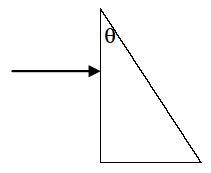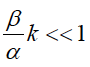# a plane wave with wave number k is progressing in a perpendicular fashion towards a triangular prism, made up of one straight angle and a top angle designated as θ (see illustration). a) assume that the refraction index outside of the prism is equal to 1, while inside the prism it is n. Please draw out the ray's trajectory, and calculate the change in angle between the incoming ray and the outgoing ray as a result of its trajectory through the prism. b) it is given that outside of the prism the wave satisfies the dispersion relation

Question-AnswerCategory: Physicsa plane wave with wave number k is progressing in a perpendicular fashion towards a triangular prism, made up of one straight angle and a top angle designated as θ (see illustration). a) assume that the refraction index outside of the prism is equal to 1, while inside the prism it is n. Please draw out the ray's trajectory, and calculate the change in angle between the incoming ray and the outgoing ray as a result of its trajectory through the prism. b) it is given that outside of the prism the wave satisfies the dispersion relationa plane wave with wave number k is progressing in a perpendicular fashion towards a triangular prism, made up of one straight angle and a top angle designated as θ (see illustration).
a) assume that the refraction index outside of the prism is equal to 1, while inside the prism it is n. Please draw out the ray’s trajectory, and calculate the change in angle between the incoming ray and the outgoing ray as a result of its trajectory through the prism.
b) it is given that outside of the prism the wave satisfies the dispersion relation ω(k)=αoutk+βoutk2, while inside of the prism it satisfies ω(k)=αink+βink2.
i) while neglecting dispersion, what is this material’s refractive index? what will be the wave-number inside the prism?
ii) for αout=αin=α, obtain the dependence of refractive index n(k) on incoming wave-number k. Simplify the expression that you obtained by assuming long waves, that is to say:iii) building on the previous paragraph (and under the same assumptions): if a wave-package of width Δk (the difference between the minimal and the maximal wave number in the package) hits the prism, what will be the maximum difference in dispersion angles between the components of the ray? Express your answer though Δk, α, βin, βout​, θ.